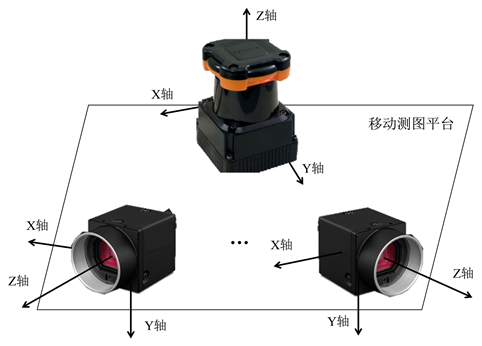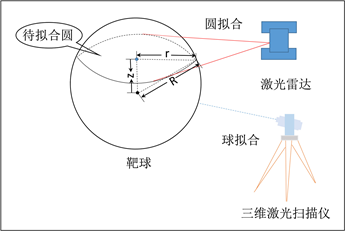# 室内移动测图平台的多传感器联合标定Extrinsic Calibration of Multi LiDAR-Camera Robotic System for Indoor Mapping

DOI: 10.12677/GST.2019.74021, PDF, 下载: 465  浏览: 933  国家自然科学基金支持

Abstract: Multi LiDAR-camera is integrated on the indoor mapping robotic system for mission of high-precision positioning and three-dimensional spatial data acquisition. Accurate extrinsic calibration of sensors is the basis of effective utilization of multi-sensors data, and is also one of key issues of indoor mobile mapping research. This paper presents a convenient calibration method for multi-sensors. First, some control points are laid on the body of the robot to create the robot body coordinate system. Then, several identifiable targets are fixed in the effective field of view of LiDAR and camera. The coordinate value of targets in the robot body coordinate system is obtained by three-dimensional laser scanner. Finally, EPNP algorithm is used to calculate the extrinsic of camera based on the corresponding relationship between two-dimensional points and three-dimensional points, and RANSAC circle fitting is used to calibrate the 2D LiDAR. Experiments show that the camera reprojection error is less than 1 pixel and the calibration result of 2D LiDAR is better than 1 cm.

1. 引言

2. 传感器联合标定方法Figure 1. Flow chart of extrinsic calibration for Indoor Mapping Robot

2.1. 坐标系定义及坐标系转换参数计算Figure 2. Schematic diagram of sensor coordinate system

1) 相机坐标系：以相机的投影中心为坐标原点，X轴向右，Y轴向下，Z轴向右构成笛卡尔右手坐标系，使用 ${p}_{i}^{c}\left({x}_{i}^{c},{y}_{i}^{c},{z}_{i}^{c}\right)$ 表示相机坐标系下坐标。

2) 扫描仪坐标系：以扫描仪的激光发射中心为坐标原点，X轴向右，Y轴向前，Z轴向上构成笛卡尔右手坐标系，使用 ${p}_{i}^{l}\left({x}_{i}^{l},{y}_{i}^{l},{z}_{i}^{l}\right)$ 表示扫描仪坐标系下坐标。

3) 机身坐标系：为自定义坐标系，其中X轴向右，Y轴向前，Z轴向上构成笛卡尔右手坐标系，在文中使用 ${p}_{i}^{b}\left({x}_{i}^{b},{y}_{i}^{b},{z}_{i}^{b}\right)$ 表示该坐标系下坐标系。

${p}_{i}^{b}=R\left({A}_{x},{A}_{y},{A}_{z}\right)\left[\begin{array}{c}\begin{array}{c}{x}_{i}^{l}\\ {y}_{i}^{l}\\ {z}_{i}^{l}\end{array}\end{array}\right]+\left[\begin{array}{c}\begin{array}{c}{t}_{1}^{l}\\ {t}_{2}^{l}\\ {t}_{3}^{l}\end{array}\end{array}\right]$ (1)

$R=\left[\begin{array}{ccc}\mathrm{cos}{A}_{z}& -\mathrm{sin}{A}_{z}& 0\\ -\mathrm{sin}{A}_{z}& \mathrm{cos}{A}_{z}& 0\\ 0& 0& 1\end{array}\right]\left[\begin{array}{ccc}\mathrm{cos}{A}_{y}& 0& -\mathrm{sin}{A}_{y}\\ 0& 1& 0\\ -\mathrm{sin}{A}_{y}& 0& \mathrm{cos}{A}_{y}\end{array}\right]\left[\begin{array}{ccc}1& 0& 0\\ 0& \mathrm{cos}{A}_{x}& \mathrm{sin}{A}_{x}\\ 0& -\mathrm{sin}{A}_{x}& \mathrm{cos}{A}_{x}\end{array}\right]$ (2)

2.2. 相机外参数标定

${p}_{j}^{l}={\sum }_{i=1}^{4}{W}_{i,j}{c}_{i}^{pb}$ (3)

${p}_{j}^{c}={\sum }_{i=1}^{4}{W}_{i,j}{c}_{i}^{pc}$ (4)

${z}_{i}^{c}\left[\begin{array}{c}{u}_{i}\\ {v}_{i}\\ 1\end{array}\right]=\left[\begin{array}{ccc}{f}_{u}& 0& {u}_{c}\\ 0& {f}_{v}& {v}_{v}\\ 0& 0& 1\end{array}\right]\left[\begin{array}{c}{x}_{i}^{c}\\ {y}_{i}^{c}\\ {z}_{i}^{c}\end{array}\right]$ (5)

${f}_{u}$${f}_{v}$ 为相机焦距， ${u}_{c}$${v}_{v}$ 为主点坐标。结合公式(4)，则公式(5)可以表示成：

${z}_{i}^{c}\left[\begin{array}{c}{u}_{i}\\ {v}_{i}\\ 1\end{array}\right]=\left[\begin{array}{ccc}{f}_{u}& 0& {u}_{x}\\ 0& {f}_{c}& {v}_{y}\\ 0& 0& 1\end{array}\right]{\sum }_{i=1}^{4}{W}_{i,j}\left[\begin{array}{c}{x}_{i}^{pc}\\ {y}_{i}^{pc}\\ {z}_{i}^{pc}\end{array}\right]$ (6)

$\left\{\begin{array}{l}{\sum }_{j=1}^{4}{W}_{i,j}{f}_{u}{x}_{i}^{pc}+{W}_{i,j}\left({u}_{c}-{u}_{i}\right){z}_{i}^{c}=0\\ {\sum }_{j=1}^{4}{W}_{i,j}{f}_{u}{x}_{i}^{pc}+{W}_{i,j}\left({u}_{c}-{u}_{i}\right){z}_{i}^{c}=0\end{array}$ (7)

2.3. 扫描仪外参数标定Figure 3. Extrinsic calibration of 2D LiDAR

$|{Z}_{l}|=\sqrt{{R}^{2}-{r}^{2}}$ (8)

${x}^{2}+{y}^{2}+{z}^{2}-Ax-By-Cz+D=0$ (9)

$\left[\begin{array}{cccc}{x}_{1}& {y}_{1}& {z}_{1}& -1\\ ⋮& ⋮& ⋮& ⋮\\ {x}_{n}& {y}_{n}& {z}_{n}& -1\end{array}\right]\left[\begin{array}{c}A\\ B\\ C\\ D\end{array}\right]=\left[\begin{array}{c}{x}_{1}^{2}+{y}_{1}^{2}+{z}_{1}^{2}\\ ⋮\\ {x}_{n}^{2}+{y}_{n}^{2}+{z}_{n}^{2}\end{array}\right]$ (10)

$\left[\begin{array}{c}A\\ B\\ C\\ D\end{array}\right]={\left[\begin{array}{cccc}\sum {x}_{i}^{2}& \sum {x}_{i}{y}_{i}& \sum {x}_{i}{z}_{i}& -\sum {x}_{i}\\ \sum {x}_{i}{y}_{i}& \sum {x}_{1}^{2}& \sum {y}_{i}{z}_{i}& -\sum {y}_{i}\\ \sum {x}_{i}{z}_{i}& \sum {y}_{i}{z}_{i}& \sum {x}_{1}^{2}& -\sum {z}_{i}\\ -\sum {x}_{i}& -\sum {y}_{i}& -\sum {z}_{i}& n\end{array}\right]}^{-1}\left[\begin{array}{c}\sum {x}_{i}\left({x}_{i}^{2}+{y}_{i}^{2}+{z}_{i}^{2}\right)\\ \sum {y}_{i}\left({x}_{i}^{2}+{y}_{i}^{2}+{z}_{i}^{2}\right)\\ \sum {z}_{i}\left({x}_{i}^{2}+{y}_{i}^{2}+{z}_{i}^{2}\right)\\ \sum -\left({x}_{i}^{2}+{y}_{i}^{2}+{z}_{i}^{2}\right)\end{array}\right]$ (11)

3. 实验与结果分析Table 1. Intrinsic parameters of cameraFigure 4. Experiment of camera extrinsic calibration, (a) Relationship between Pixel Error and Attitude solution accuracy; (b) Relationship between Pixel Error and Position solution accuracy; (c) Relationship between Pixel Error and Reprojection error; (d) Reprojection error of image control pointsFigure 5. Experiment of 2D LiDAR extrinsic calibration, (a) Data interpretation of 2D LiDAR; (b) RANSAC circle fittingTable 2. Coordinate of common points in 2D LiDAR extrinsic calibration experimentTable 3. Experimental results of extrinsic calibration

4. 总结与分析

NOTES

*通讯作者。

  Cortes, C., Shahbazi, M. and Ménard, P. (2018) UAV-LiCAM System Development: Calibration and Geo-Referencing. The Interna-tional Archives of the Photogrammetry, Remote Sensing and Spatial Information Sciences, Karlsruhe, 10-12 October 2018, 107-114. https://doi.org/10.5194/isprs-archives-XLII-1-107-2018  张靖, 江万寿. 激光点云与光学影像配准:现状与趋势[J]. 地球信息科学学报, 2017, 19(4): 528-539.  Liu, H., Da, F.P. and Gai, S.Y. (2019) Research on Multi-Camera Calibration and Point Cloud Correction Method Based on Three-Dimensional Calibration Object. Optics and Lasers in Engineering, 115, 32-41. https://doi.org/10.1016/j.optlaseng.2018.11.005  Geiger, A., Moosmann, F., Car, O. and Schuster, B. (2012) Automatic Camera and Range Sensor Calibration Using a Single Shot. Proceedings IEEE International Conference on Robotics and Automation, St Paul, 14-18 May 2012, 3936-3943. https://doi.org/10.1109/ICRA.2012.6224570  Bujnak, M., Kukelova, Z. and Pajdla, T. (2008) A General Solution to the P4P Problem for Camera with Unknown Focal Length. IEEE Computer Society Conference on Computer Vision and Pattern Recognition, Anchorage, 24-26 June 2008, 1-8. https://doi.org/10.1109/CVPR.2008.4587793  Lepetit, V., et al. (2009) EPnP: An Accurate O(n) Solution to the PnP Prob-lem. International Journal of Computer Vision, 81, 155-166. https://doi.org/10.1007/s11263-008-0152-6  张慧娟, 熊芝, 等. 基于EPNP算法的单目视觉测量系统研究[J]. 红外与激光工程, 2018, 48(5): 190-195.  闫利, 曹亮, 陈长军, 等. 车载全景影像与激光点云数据配准方法研究[J]. 测绘通报, 2015(3): 32-36.  李明磊, 高欣圆, 李广云, 等. 地面三维激光扫描仪与外架数码相机间安置参数的高精度标定[J]. 光学精密工程, 2016, 24(9): 2158-2166.  姚连璧, 汪志飞, 孙海丽. 车载激光扫描仪外参数标定方法设计与实现[J]. 同济大学学报(自然科学版), 2015, 44(1): 161-166.  Zhang, Q. and Pless, R. (2005) Extrinsic Calibration of a Camera and Laser Range Finder (Improves Camera Calibration). IEEE/RSJ International Conference on Intelligent Robots & Systems, Sendai, 28 September-2 October 2004, 2301-2306.  Dong, W. and Isler, V. (2018) A Novel Method for the Extrinsic Calibration of a 2D Laser Rangefinder and a Camera. IEEE Sensors Journal, 18, 4200-4211. https://doi.org/10.1109/JSEN.2018.2819082  Zhang, Z. (2000) A Flexible New Technique for Camera Calibration. IEEE Transactions on Pattern Analysis and Machine Intelligence, 22, 1330-1334.